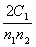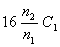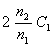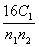Tuesday 29th September 2020

# AIPMT 2010 Physics

Q.1. Consider the following two statements:

1. Kirchhoff's junction law follows from the conservation of charge.
2. Kirchhoff's loop law follows from the conservation of energy

Which of the following is correct?

1. Both (A) and (B) are wrong
2. (A) is correct and (B) is wrong
3. (A) is wrong and (B) is correct
4. (4) Both (A) and (B) are correct

Sol:

Junction − conservation of charge Loop − conservation of energy

Q. 2. Electromagnets are made of soft iron because soft iron has:

1. low retentivity and high coercive force
2. high retentivity and high coercive force
3. low retentivity and low coercive force
4. high retentivity and low coercive force

Q. 3.  A series combination of n1 capacitors, each of value C1, is charged by a source of
potential difference 4 V. When another parallel combination of n2 capacitors, each
of value C2, is charged by a source of potential difference V, it has the same (total)
energy stored in it, as the first combination has. The value of C2, in terms of C1, is then:

1.2.3.4.Sol: